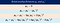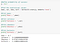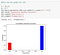# Bernoulli Distribution — Probability Tutorial with Python

## Bernoulli distribution tutorial — diving into the discrete probability distribution of a random variable with examples in Python

Author(s): Pratik Shukla, Roberto Iriondo

Last updated on September 25, 2020.

In this series of tutorials, we will dive into probability distributions in detail. We will not just showcase formulas, but instead, we will see how each of the formulas derive from their basic definitions (as it is essential to understand the math behind the derivations), and we will showcase such by using some examples in Python.

This tutorial’s code is available on Github and its full implementation as well on Google Colab.

📚 Check out our Moment Generating Function Tutorial with Python. 📚

Before diving deep into probability distributions, let’s first understand some basic terminology about a random variable.

# What is a Random Variable?

A variable is called a random variable if its value is unknown. In other words, a variable is a random variable if we cannot get the same variable using any kind of function.

A random variable is a variable whose possible values are numerical outcomes of a random phenomenon.

Properties of a random variable:

Examples:

In figure 1, we show that the outcome is not dependent on any other variables. So the output of tossing a coin will be random.

2. Rolling a fair die:

In figure 2, we can notice that the output of a die cannot be predicted in advance, and it is not dependent on any other variables. So we can say that the output will be random.

Now let’s have a brief look at non-random variables.

In the example above, we can see that in example 1, we can quickly get the value of variable x by subtracting one from both sides. Therefore, the value of x is not random, but it is fixed. In the second example, we can see that the value of variable y is dependent on the value of variable x, where we can notice that the value of y changes according to the value of x. We can generate the same output variable y when we plugin the same value of x. So variable y is not random at all. In probability distributions, we will work with random variables.

# Discrete Random Variable:

A random variable is called a discrete random variable if its values can be obtained by counting. Discrete variables can be counted a finite amount of time. The critical thing to note here is that discrete variables need not be an integer. We can have discrete random variables that are finite float values.

Examples:

# Continuous Random Variable:

A random variable is called a continuous random variable if its values can be obtained by measuring. We cannot count continuous variables in a finite amount of time. In other ways, we can say that it will take an infinite amount of time to count continuous variables.

Examples:

The vital thing to notice is that we are mentioning the word “Exact” here. It means that all the measurements we take are up to absolute precision.

For example, if we measure the completion time of a race for an athlete, we can say that he completed the race in 9.5 seconds. To be more precise, we can say that he completed the race in 9.52 seconds. To be more precise, we can say that the athlete completed the race in 9.523 seconds. To add more precision to the time taken, we can also say that he completed the race in 9.5238 seconds. If we keep on doing this, we can take this thing to an infinite level of precision, and it will take us an infinite amount of time to measure it. That is why it is called a continuous variable.

# Main Difference Between Discrete and Continuous Variable:

Example: What is your current age?

The example is classified into the group of continuous variables. As discussed above, we can say the following about your age:

Notice that we can continue writing age with more and more precision. Therefore, we can not count the exact age of a person in a finite amount of time. That is why it is a continuous variable.

On the other hand, if the question was, what is your current age in years?”. Then, in this case, the variable can be classified in the group of discrete variables. Since we already know that my age at this point is an “X amount of years.”

Next, let’s discuss Probability Distributions. Probability distributions are bases on data types, and they can be either Discrete or Continuous.

# Probability Distribution:

A probability distribution is a mathematical function that gives the probabilities of occurrence of different possible outcomes for an experiment.

# Bernoulli Distribution:

## Conditions for the Bernoulli Distribution

If our data satisfies the conditions above, then:

A discrete random variable X follows a Bernoulli distribution with the probability of success=p.
Visual representation of Bernoulli distribution:

Examples:

For instance:

There are only two candidates in an election: Patrick and Gary, and we can either vote for Patrick or Gary.

• P(Success) = P(1) = Vote for Patrick = 0.7
• P(Failure) = P(0) = Vote for Gary = 0.3

Here we have only one trial and only two possible outcomes. So we can say that the data follows a Bernoulli distribution. To visualize it:

# Probability Mass Function (PMF):

A probability mass function of a discrete random variable X assigns probabilities to each of the possible values of the random variable. By using PMF, we can get the probabilities of each random variable. 

Let X be a discrete random variable with its possible values denoted by x1, x2, x3, …, xn. The probability mass function(PMF) must satisfy the following conditions:

Properties of PMF:

2. All the possible probability values must be greater than or equals to 0.

## Probability Mass Function (PMF) for Bernoulli Distribution:

Let’s visualize the function:

# Mean for Bernoulli Distribution:

The mean of discrete random variable X is it is a weighted average. Its probability weights each value of random variable X. In the Bernoulli Distribution, the random variable X can take only two values: 0 and 1, and we can quickly get the weight by using the Probability Mass Function(PMF).

Mean: The mean of a probability distribution is the long-run arithmetic average value of a random variable having that distribution.

The expected value E[X] expresses the likelihood of the favored event.

The expected value or the mean of Bernoulli Distribution is given by:

Mean of Bernoulli Distribution:

# Variance for Bernoulli Distribution:

Variance(σ2) is the measure of how far each number from the set of random numbers is from the mean. The square root of the variance is called the standard deviation.

Based on its definition:

The variance of a discrete probability distribution:

In our case, variable x can take only two values: 0 and 1.

The variance of Bernoulli Distribution:

There is a more popular form to find variance in statistics:

Let’s see how this came into existence.

Basically, the variance is the expected value of the squared difference between each value and the mean of the distribution. 

From the definition of variance, we can then:

Finding the variance using this formula:

In figure 25, we can see that the Bernoulli distribution variance is the same regardless of which formula we use.

# Standard Deviation for Bernoulli Distribution:

A standard deviation is a number used to tell how measurements for a group are spread out from the average (mean or expected value) .

A low standard deviation means that most of the numbers are close to the average, while a high standard deviation means that the numbers are more spread out.

# Mean Deviation for Bernoulli Distribution:

The mean deviation is the mean of the absolute deviations of a data set about the data’s mean.

Based on the definition:

For Discrete probability Distribution:

Finding the mean deviation for the Bernoulli distribution:

# Moment Generating Function For Bernoulli Distribution:Figure 30; Summary of the relationship between central and raw moments.

For the following derivations, we will use the formulas we derived in our previous tutorial. So we recommend you to check out our tutorial on Moment Generating Function.

Moment Generating Function:

## 1. First Moment:

a. First Raw Moment:

## 2. Second Moment:

a. Second Raw Moment:

b. Second Central Moment (Variance):

## 3. Third Moment:

a. Third Raw Moment:

b. Third Central Moment:

c. Third Standardized Moment: (Skewness)

## 4. Fourth Moment:

a. Fourth Raw Moment:

b. Fourth Centered Moment:

c. Fourth Standardized Moment:( Kurtosis):

# Cumulative Distribution Function(CDF):

Based on the Probability Mass Function (PMF), we can write the Cumulative Distribution Function (CDF) for the Bernoulli distribution as follows:

Next to the fun part, let’s move on to its implementation in Python.

# Python Implementation:

2. Find the moments:Figure 46: Finding the moments for the Bernoulli distribution with a p-value of 0.7.

3. Get the mean value:

4. Get median value:

5. Get variance value:

6. Get standard Deviation value:

7. Probability Mass Function (PMF):

8. Plotting the PMF:

9. Cumulative Density Function (CDF):

10. Plot the CDF:

11. Plot the bar graph for PMF:

12. Plot the bar graph for CDF:Figure 56: The bar graph of the CDF for p-value 0.7.

13. Output for different experiments:

# Summary of the Bernoulli Distribution:

That is it for the Bernoulli distribution tutorial. We hope you enjoyed reading it and learned something new. We will try to cover more probability distributions in-depth in the future. Any suggestions or feedback is crucial to continue to improve. Please let us know in the comments if you have any.

DISCLAIMER: The views expressed in this article are those of the author(s) and do not represent the views of Carnegie Mellon University, nor other companies (directly or indirectly) associated with the author(s). These writings do not intend to be final products, yet rather a reflection of current thinking, along with being a catalyst for discussion and improvement.

Published via Towards AI

# References:

 Probability Distribution, Wikipedia, https://en.wikipedia.org/wiki/Probability_distribution

 Bernoulli Distribution, Statlect, https://www.statlect.com/probability-distributions/Bernoulli-distribution

 Variance, Wikipedia, https://en.wikipedia.org/wiki/Variance

 Bernoulli Distribution, Wikipedia, https://en.wikipedia.org/wiki/Bernoulli_distribution

 Bernoulli Distribution, SciPy.org, https://docs.scipy.org/doc/scipy-0.14.0/reference/generated/scipy.stats.bernoulli.html

 Probability Mass Function, Wikipedia, https://en.wikipedia.org/wiki/Probability_mass_function

 Mean and Variance of Probability Distributions, Probabilistic World, https://www.probabilisticworld.com/mean-variance-probability-distributions/

 Standard Deviation, Wikipedia, https://en.wikipedia.org/wiki/Standard_deviation

## Towards AI

### By Towards AI

Towards AI publishes the best of tech, science, and engineering. Subscribe with us to receive our newsletter right on your inbox. For sponsorship opportunities, please email us at pub@towardsai.net Take a look

Written by

Written by

## Towards AI Team

#### Publishing the Best of Tech, Science, and Engineering | Editorial → https://towardsai.net/p/editorial | Subscribe→ https://towardsai.net/subscribe — @Towards_AI